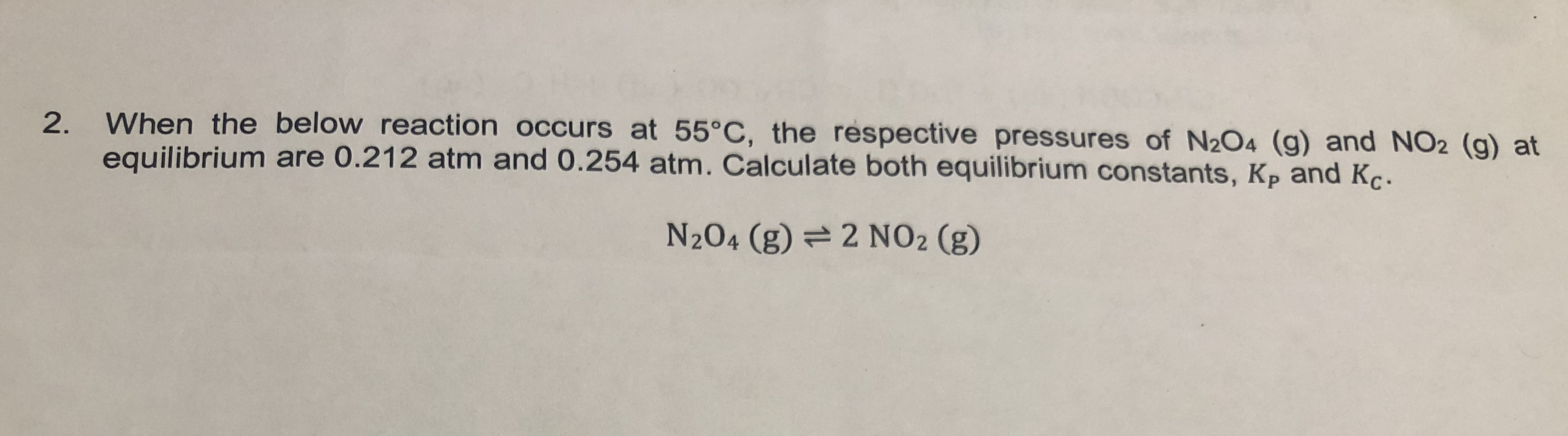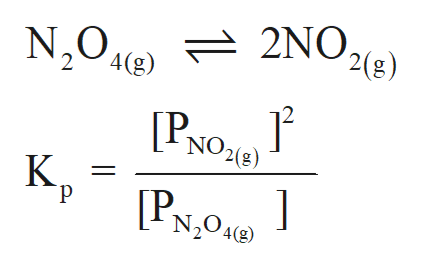When the below reaction occurs at 55°C, the respective pressures of N2O4 (g) and NO2 (g) at2.equilibrium are 0.212 atm and 0.254 atm. Calculate both equilibrium constants, Kp and KçN2O4 (g)2 NO2 (g)

Questionhelp_outlineImage TranscriptioncloseWhen the below reaction occurs at 55°C, the respective pressures of N2O4 (g) and NO2 (g) at 2. equilibrium are 0.212 atm and 0.254 atm. Calculate both equilibrium constants, Kp and Kç N2O4 (g) 2 NO2 (g) fullscreen
Step 1

A reversible chemical reaction can move in either forward or backward direction. The stage of a reversible reaction at which the rate of forward and backward reaction become equal to each other is called an equilibrium stage.

Step 2

The equilibrium constant for a reaction is the ratio of equilibrium concentration of product and reactant. If the reaction is given in gaseous state, then the equilibrium pressure must be considered.

Step 3

The Kp expression for the below ...help_outlineImage TranscriptioncloseN2042) 2NO, '2(g) '4(g) 2 NO2(8) [PNO К. р [P N204() fullscreen

Want to see the full answer?

See Solution

Want to see this answer and more?

Our solutions are written by experts, many with advanced degrees, and available 24/7

See Solution
Tagged in

Equilibrium Concepts